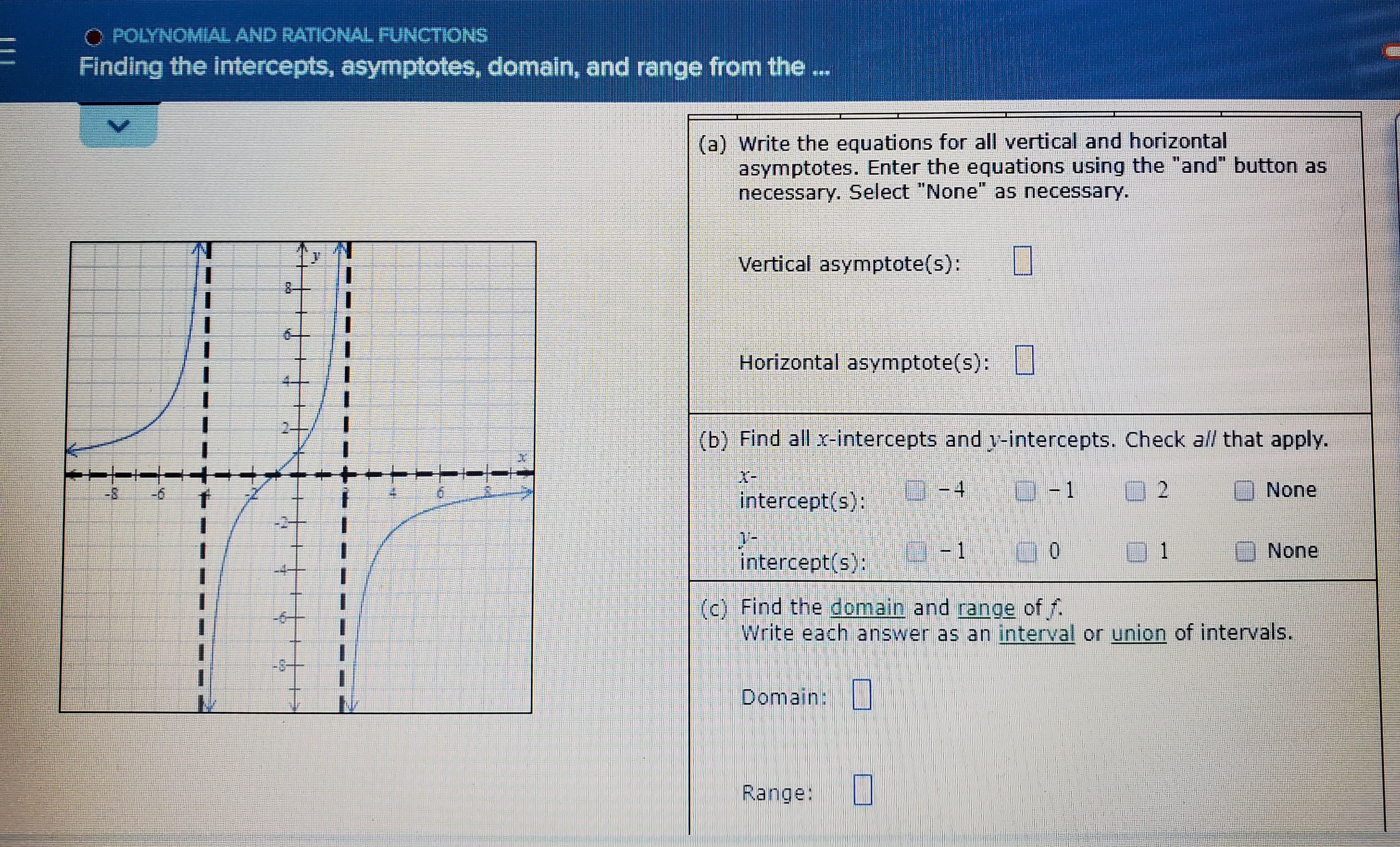# O POLYNOMIAL AND RATIONAL FUNCTIONS Finding the intercepts, asymptotes, domain, and range from the (a) Write the equations for all vertical and horizontal asymptotes. Enter the equations using the "and" button as necessary. Select "None" as necessary. Vertical asymptote(s): Horizontal asymptote(s): (b) Find all x-intercepts and y-intercepts. Check all that apply. -4 1 None 2 intercept(s): None 0 1 intercept(s): (c) Find the domain and range of f. Write each answer as an interval or union of intervals. -6 Domain: Range:

Question

Finding the intercepts, asymptotes, domain and range of the attached problemhelp_outlineImage TranscriptioncloseO POLYNOMIAL AND RATIONAL FUNCTIONS Finding the intercepts, asymptotes, domain, and range from the (a) Write the equations for all vertical and horizontal asymptotes. Enter the equations using the "and" button as necessary. Select "None" as necessary. Vertical asymptote(s): Horizontal asymptote(s): (b) Find all x-intercepts and y-intercepts. Check all that apply. -4 1 None 2 intercept(s): None 0 1 intercept(s): (c) Find the domain and range of f. Write each answer as an interval or union of intervals. -6 Domain: Range: fullscreen

### Want to see this answer and more?

Experts are waiting 24/7 to provide step-by-step solutions in as fast as 30 minutes!*